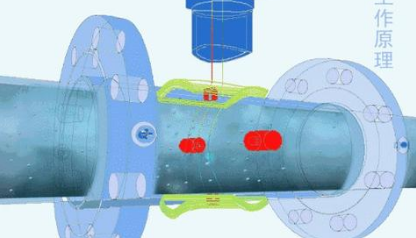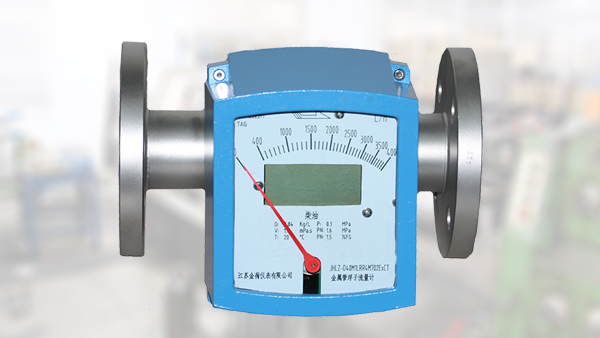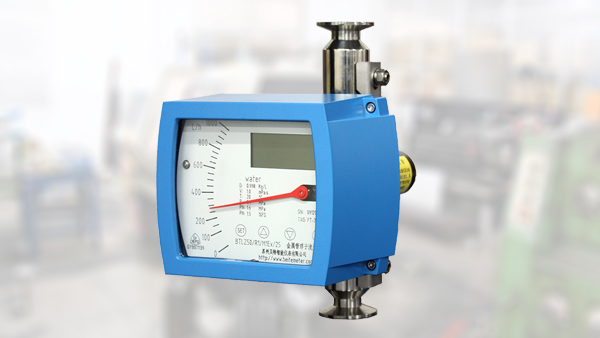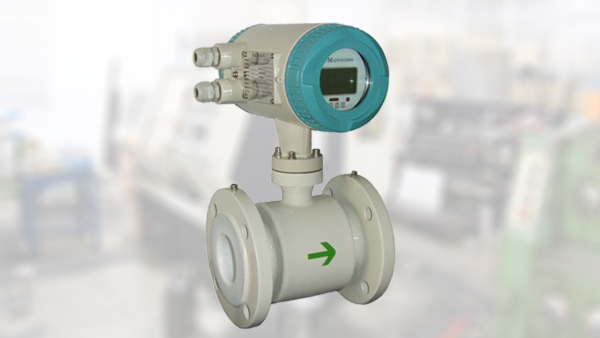# 转子流量计选型的五个重要因素「选型举例」1.流量计的性能要求;

2.流体特性;

3.安装要求;

4.环境条件;

5.流量计的价格。

## 转子流量计选型举例

(1)氧气密度

ρ1=ρ20×(P1T20/PNT1Z)=1.331×{(1.3+0.1)×(273.15+20)/[0.1013×(273.15+30)×0.992]}

=17.93kg/m3

(2)实际流量：

Qs=Q20ρ20/ρ

Qsmax=Q20maxρ20/ρ1=110×1.331/17.93=8.166

Qsmin=Q20minρ20/ρ1=10×1.331/17.93=0.742

(3)由金属转子流量计实际使用流体状况下的流量修正公式：

QNmax=Qsmax/0.2696=8.166/0.2696=30.29

QNmin=Qsmax/0.2696=0.742/0.2696=2.75

【相关推荐】### 如何正确操作金属管浮子流量计？智能仪表厂家告诉您### 金属管浮子流量计应用领域有哪些？400-839-1983
• 400电话

400-839-1983